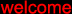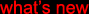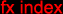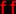(c) 2000-2009

mode zero

boss dm-100 and dm-300 delay machines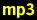snare A, no effectdm-100: snare A, rate = 5, intensity = 6, echo vol = maxdm-100: snare A, rate = 6, intensity = 6, echo vol = maxdm-100: snare A, rate = 8, intensity = 4, echo vol = maxsnare B, no effectdm-100: snare B, rate = 5, intensity = 5, echo vol = maxdm-100: snare B, rate = 8, intensity = 5, echo vol = maxdm-100: snare B, rate = 3, intensity = 5 --> 8, echo vol = maxdm-100: snare B, rate = 7, intensity = 2, echo vol = max